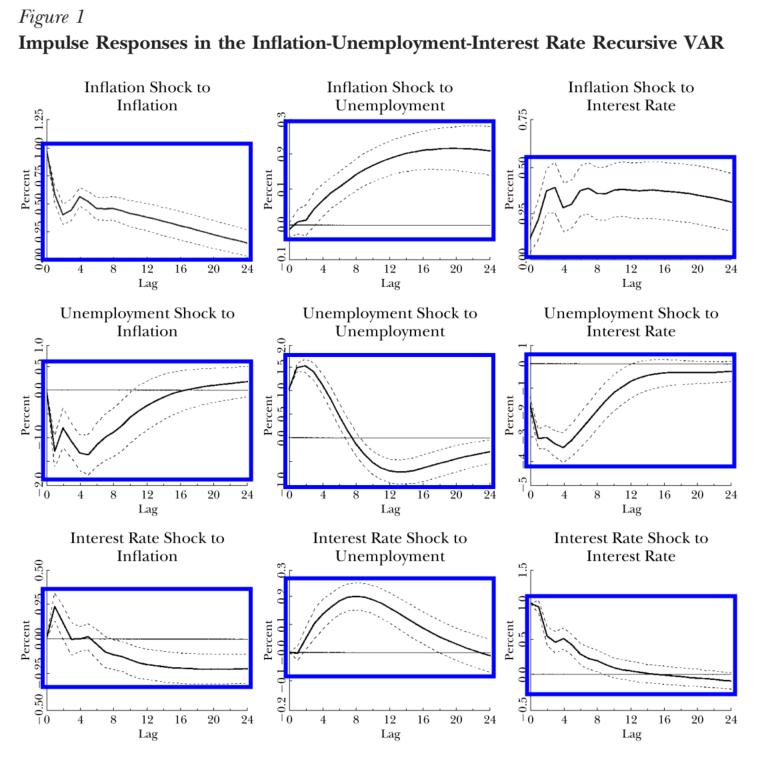# #StackBounty: #statistical-significance #vector-autoregression #impulse-response Quantifying the significance of impulse response funct…

### Bounty: 50

I use Stock and Watson’s classic reference on vector autoregressions for this question. They carry out a VAR on inflation, unemployment and the interest rate and thereby produce the following matrix of impulse response functions (IRFs).I would like to understand if there is a way to quantify the significance of these IRFs. To be more precise, each plot shows a function of lag (time from shock) which takes some asymptotic value, call it $$x_infty$$, to which is also assigned some asymptotic standard error, call it $$sigma_infty$$ (I believe that the error bars in the figure are the standard 95% confidence interval, i.e. $$1.96sigma_l$$ where $$sigma_l$$ is the standard error at lag $$l$$.) Is there a meaningful way to combine $$x_infty$$ and $$sigma_infty$$ to produce a measure of statistical significance? In other words, how can one numerically capture the intuitive sense in which an IRF with thick error bands is "less significant" than one in which the error bands are slimmer?

(A further example of what I am trying to accomplish, in case the above isn’t clear: in the unrelated procedure of least-squares regression, one divides a coefficient-estimate $$beta$$ by its standard error to produce a $$t$$-statistic. Is something similar available here?)

Get this bounty!!!

This site uses Akismet to reduce spam. Learn how your comment data is processed.